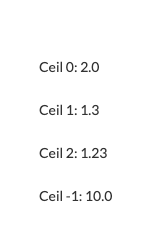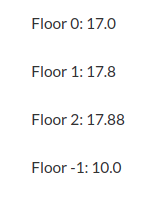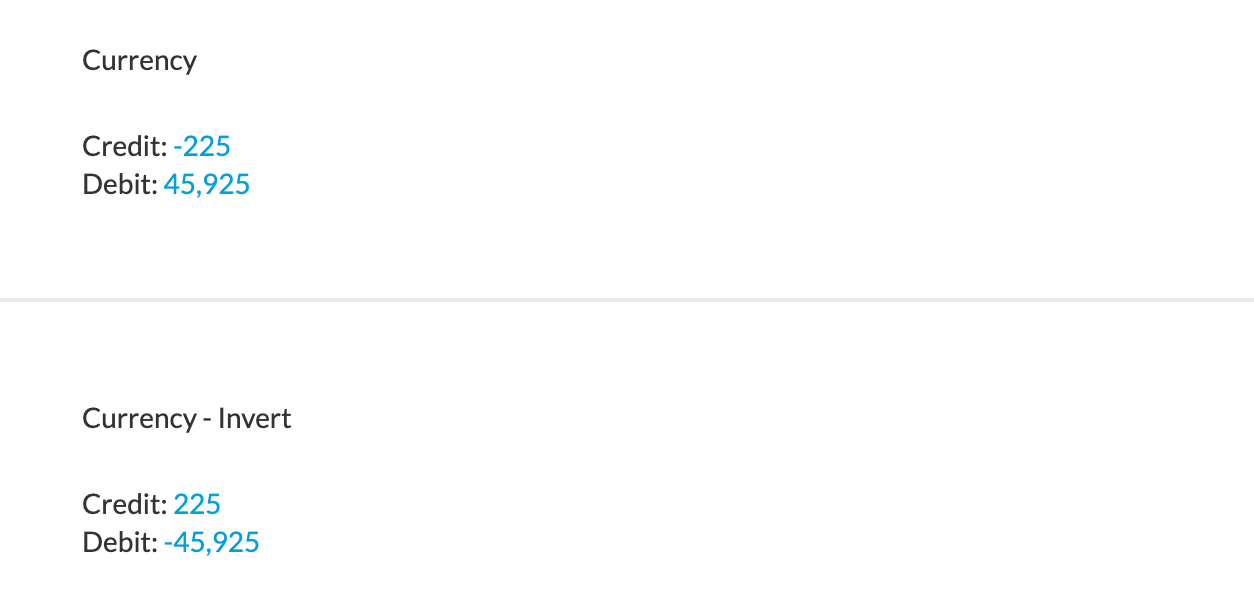# Number

## Abs

Outputs a number into an absolute value.

``````{{ -23 | abs }}
``````

This filter can also be applied with the following syntax.

``````{{ ABS(-23) }}
``````
Output
23

## Ceil

Allows to round the input UP. For example, the nearest whole number of 1.2 is 1, however, if you use ceil:0 filter, it will round UP the value and the result will be 2.

🚧

Ceil filter needs to be used with precision for it to work.

Depending on the number used with ceil, the value can be rounded UP in a different way, for example:
ceil:0 is to the nearest whole number
ceil:1 is to the nearest 0.1
ceil:2 is to the nearest 0.01
ceil:-1 is to the nearest 10

``````Ceil 0: {{ 1.2222 |ceil:0 }}

Ceil 1: {{ 1.2222 |ceil:1 }}

Ceil 2: {{ 1.2222 |ceil:2 }}

Ceil -1: {{ 1.2222 |ceil:-1 }}
``````
Output## Floor

Similar to `ceil`, this allows to round the input DOWN. For example, the nearest whole number of 1.8 is 2, however, if you use floor:0 filter, it will round DOWN the value and the result will be 1.

🚧

Floor filter needs to be used with precision for it to work.

Depending on the number used with floor, the value can be rounded DOWN in a different way, for example:
floor:0 is to the nearest whole number
floor:1 is to the nearest 0.1
floor:2 is to the nearest 0.01
floor:-1 is to the nearest 10

``````Floor 0: {{ 17.8888 | floor:0 }}

Floor 1: {{ 17.8888 | floor:1 }}

Floor 2: {{ 17.8888 | floor:2 }}

Floor -1: {{ 17.8888 | floor:-1 }}
``````
Output## Currency

This will output a value into the currency format, depending on the chosen language in Silverfin. This is a filter made by Silverfin.

🚧

"#61" will give the value of all 61-accounts for the selected period.

``````{{ #61 | currency }}
``````
Output
121,590.31

## Invert

Invert how the value of accounts are displayed based on their type.

This filter will only work together with the 'currency' filter

``````Currency

Credit: {{ accounts_c | currency:0 }}
Debit: {{ accounts_d | currency:0 }}

Currency - Invert

Credit: {{ accounts_c | currency:0, invert:true }}
Debit: {{ accounts_d | currency:0, invert:true }}
``````
Output## Integer

This will output a value without decimals.

❗️

Please note that an integer returns a formatted string, not an actual number. This behaviour can be misleading when making calculations because of the commas and dots.

``````{{ 30.99 |integer }}
``````
Output
31

Using as an integer in the following syntax will give you a different output.

``````{{ INT(30.99) }}
``````
Output
30

## Percentage

This will output a value as a percentage.

📘

The formula (#60+#70)/#70 is the calculation of gross margin, where the 60-accounts are in plus and the 70-accounts in minus.

``````{{ (#60+#70)/#70 | percentage }}
``````
Output
99.89%

## Number_to_human

This will output the value of [linkto account drop]accounts[endlinkto] into a combination of a rounded number and English word for that rounded number, if it's more than 1 thousand. This is a filter made by Silverfin.

``````{{ #6 | number_to_human }}
``````
Output
287 Thousand

## Number_to_currency

This will output a string to the currency format, depending on the language setting of Silverfin. This filter is made by Silverfin.

``````{{ "123456789.99" | number_to_currency }}
``````
Output
123.456.789,99

## Round

This will output a value to a rounded value, depending on a number that can be given as an argument. As a default, you will see two decimals after the coma.

``````Example 1 with two decimals: {{ #6 | round }}

Example 2 with zero decimals: {{ #6 | round:0 }}
``````
Output

Example 1 with two decimals: 313734.14

Example 2 with zero decimals: 313734.0

## Modulo

Divides an output by a number and returns the remainder.

``````{{ 9 | modulo:2 }}
``````
Output
1

## MAX & MIN

MAX and MIN are used to return the maximum or minimum value in a collection of data.

``````MAX: {{ MAX(30;40;50;60;70) }}
MIN: {{ MIN(30;40;50;60;70) }}
``````
Output
MAX: 70
MIN: 30

## At_least

The `at_least` filter will limit a number to a minimum value.

``````{{ 4 | at_least:5 }}
{{ 4 | at_least:3 }}
``````
Output
5
4

## At_most

The `at_most` filter will limit a number to a maximum value.

``````{{ 4 | at_most:5 }}
{{ 4 | at_most:3 }}
``````
Output
4
3